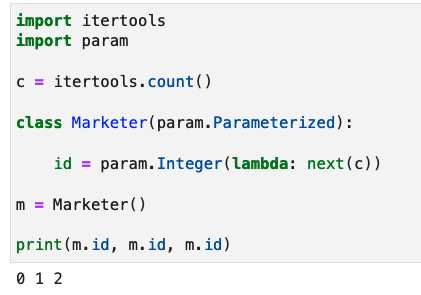# Is __init__ the equivalent of a dataclass __post_init__? binding a value via a callable

`marketer_id` is a callable that returns an ever-increasing number. I want to set the id of a new `Marketer` instance by calling `marketer_id()` when building the instance. I’m not sure if I can supply a callable as the default argument to a param and it will call it.

``````marketer_id = itertools.count()

class Marketer(Parameterized):
id = Number()   # <--- how to bind to the result of invoking marketer_id()???
front_line = Number(5)
``````

The `Number` Parameter is a subclass of the `Dynamic` parameter type that accepts a callable, this is described in more details in this section of the user guide.

``````import itertools
import param

c = itertools.count()

class Marketer(param.Parameterized):
id = param.Integer(lambda: next(c))

m = Marketer()
print(m.id, m.id, m.id)
``````But: we only want to give the object an id once at object construction time…

So a `__post_init__` or `__init__` method that binds `id` once is desired.

Oh ok. Then I guess this is one way to achieve this behavior.

``````import itertools
import param

c = itertools.count()

class Marketer(param.Parameterized):
id = param.Integer(default=0, bounds=(0, None))

def __init__(self, **params):
super().__init__(**params)
self.id = next(c)

m1 = Marketer()
print(m1.id)  # 0

m2 = Marketer()
print(m2.id) # 1
``````